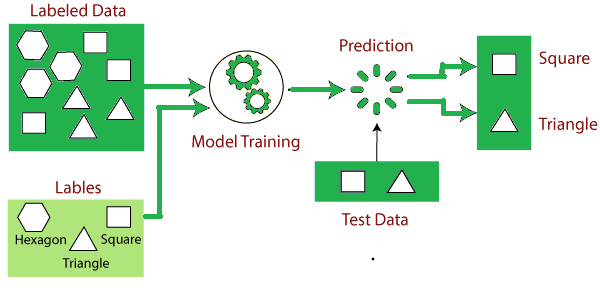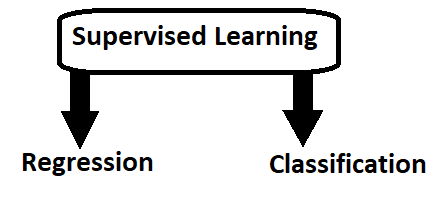# Supervised Machine Learning

In this page we will learn about What is Supervised Machine Learning?, How Supervised Learning Works?, Steps Involved in Supervised Learning, Types of supervised Machine learning Algorithms, Advantages of Supervised learning, Disadvantages of supervised learning.

## What is Supervised Machine Learning?

Supervised learning is a sort of machine learning in which machines are trained using well-labeled training data and then predict the output based on that data. The labelled data indicates that some of the input data has already been tagged with the appropriate output.

In supervised learning, the training data presented to the machines acts as a supervisor, instructing the machines on how to correctly predict the output. It uses the same notion as when a student learns under the guidance of a teacher. The process of supplying input data as well as proper output data to the machine learning model is known as supervised learning. A supervised learning algorithm's goal is to discover a mapping function that will map the input variable(x) to the output variable(y).

Supervised learning can be utilized in the real world for things like risk assessment, image categorization, fraud detection, spam filtering, and so forth.

## How Supervised Learning Works?

Models are trained using a labelled dataset in supervised learning, where the model learns about each category of input. The model is tested using test data (a subset of the training set) when the training phase is completed, and it then predicts the output.

The following example and diagram will help you understand how supervised learning works:Assume we have a dataset with a variety of forms, such as squares, rectangles, triangles, and polygons. The model must now be trained for each shape as the initial phase.

• If a given shape has four sides and all of them are equal, it is referred to as a Square .
• The supplied shape will be labeled as a triangle if it has three sides .
• When a form has six equal sides, it is referred to as a hexagon .

After training, we use the test set to put our model to the test, and the model's objective is to recognize the shape.

The system has already been trained on a variety of forms, so when it encounters a new one, it classifies it based on a number of sides and predicts the outcome.

## Steps Involved in Supervised Learning:

• First Determine the type of training dataset
• Collect/Gather the labelled training data.
• Split the training dataset into training dataset, test dataset, and validation dataset.
• Determine the input features of the training dataset, which should have enough knowledge so that the model can accurately predict the output.
• Determine the suitable algorithm for the model, such as support vector machine, decision tree, etc.
• Execute the algorithm on the training dataset. Sometimes we need validation sets as the control parameters, which are the subset of training datasets.
• Evaluate the accuracy of the model by providing the test set. If the model predicts the correct output, which means our model is accurate.

## Types of supervised Machine learning Algorithms:## 1. Regression

If there is a relationship between the input and output variables, regression procedures are applied. It's used to predict continuous variables like weather forecasting, market trends, and so on. Some popular supervised learning regression algorithms are listed below:

• Linear Regression
• Regression Trees
• Non-Linear Regression
• Bayesian Linear Regression
• Polynomial Regression

## 2. Classification

When the output variable is categorical, meaning there are two classes, such as Yes-No, Male-Female, True-False, and so on, classification methods are utilized.

Spam Filtering,

• Random Forest
• Decision Trees
• Logistic Regression
• Support vector Machines

Note: We will discuss these algorithms in detail in later chapters.